• Call Now

1800-102-2727•

# Energy in Simple Harmonic Motion - Kinetic Energy, Potential Energy, Total Energy

Have you ever swung in a pendulum swing , if yes you may observe that you have the maximum velocity at the position when you come down and zero velocity when you are at the highest point. Why is it happening? It is because of energy when you come down the swing’s energy converted into kinetic form and when you reach at the top it has potential energy.

## What is Energy?

Energy is defined as the ability to do work. Energy objects have some kind of energy either moving or at rest. As per the laws of conservation of energy, “energy cannot be created or destroyed, although it can be converted from one form to another”. The unit of energy is joule (J).

Energy has various types. In Simple harmonic motion we deal with only mechanical energy. Mechanical energy is also of two types kinetic energy and potential energy.

## Energy in Simple Harmonic Motion

When a particle is in simple harmonic motion it also possesses energy. We know that when the particle is at mean position it have maximum velocity and kinetic energy is proportional to square of velocity, so particle have maximum kinetic energy at mean position and when it is at extreme positions it has maximum restoring force means maximum ability of doing work hence maximum potential energy. Let's see mathematically how we calculate energies in simple harmonic motion.

### Kinetic Energy of Simple Harmonic Motion

Kinetic energy of an object is due to its motion.Consider a particle of mass m is in simple harmonic motion with amplitude ‘a’ along a path PQ. let's O be the mean position. Hence OA=OB=a.

Now suppose a particle is given an infinitely small displacement dx against the restoring force force F and work down to displace the particle is dw.

Then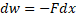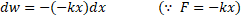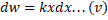Now for total work in displacing the particle from mean potion (x=0) to a distance x, will be equal to the integration of equation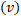.

On integrating equationwe get,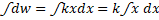Hence, Total work done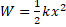Puting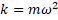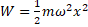The work done by external forces will store in the form of potential energy.

Therefore Potential energy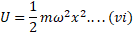From the equation we can see that Potential energy is the function of the square of x i.e.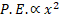. The graphical representation of Potential energy can be seen in figure.The equation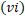can be used for calculating the Potential energy for a particle performing simple harmonic motion.

• Total energy or mechanical energy of simple harmonic motion

For a particle which is in simple harmonic motion the total energy of the particle will be equal to the sum of their kinetic and potential energy.

Total energy = kinetic energy + potential energy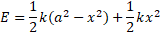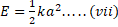We can see from the equation that the total energy of a particle in simple harmonic motion is constant. However the kinetic energy and potential energy are interchangeable. Graph of total energy with displacement is shown in figure.We can see from the graph that

• At the mean position, the total energy is in purely kinetic form. And at extreme positions the energy is in purely potential form.
• In another position kinetic energy and potential energy is interconvertible and their sum is constant.

Now consider a block connected with spring executing SHM given by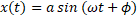Then the velocity of particle will be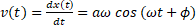SO,The kinetic energy of particle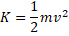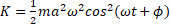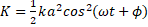From the equation we can see that kinetic energy is a function of time.

As spring force F=-kx is a conservative force so the potential energy associated with this is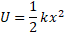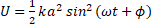Potential energy is also a function of time.

Now total energy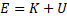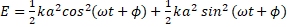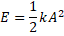The total mechanical energy of a harmonic motion is independent of time.

The graph of time K, U and E with time is shown in figure.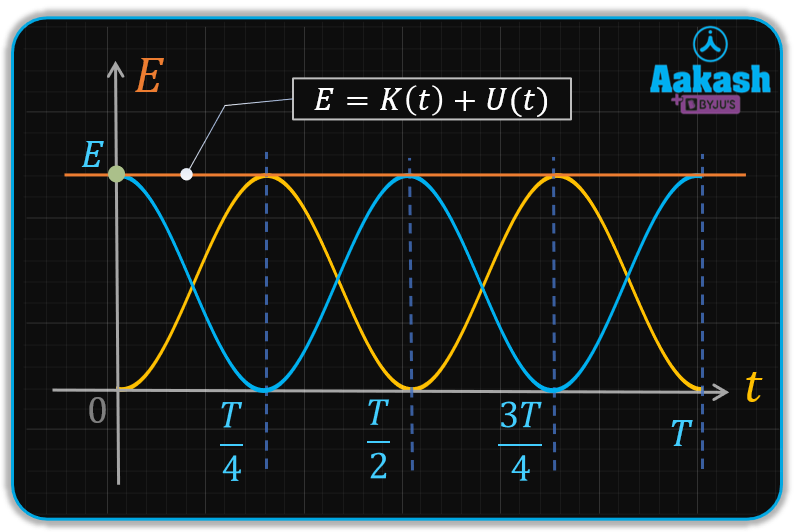## Practice Problems

Q1. A Object of mass 10 g performing simple harmonic motion of amplitude 10 cm and period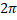s. Determine its kinetic energy when it is at a distance of 8 cm from its equilibrium position.

Ans.

Given: Mass m=10 g , Amplitude a = 10 cm , Period T =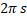, Displacement x = 8 cm

Angular velocity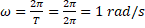Kinetic energy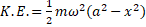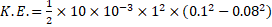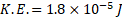Ans

Q.A particle of mass 10 g executes simple harmonic motion of amplitude 5 cm and period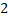s. Find Potential energy,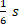After it has crossed it means potion.

A2.

Given: Mass m=10 g , Amplitude a = 5 cm , Period T =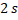, time elapsed =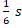, as particle passes through mean position,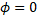.

Angular velocity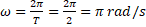Displacement of particle from mean position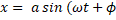)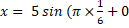)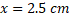Potential energy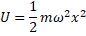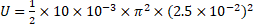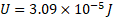Ans

Q3. Total energy of a particle in simple harmonic motion of mass 0.5 kg is 25 J. Finds its speed when crossing the mean potion.

Ans. Given: Mass m=0.5 kg , Total energy E=25 J.

When the particle passes through its mean potion it has maximum speed.

Now Total energy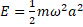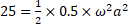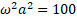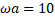But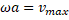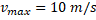Hence the speed of particles passing through the mean potion is 10 m/s.

Q4. Find the position of a particle in simple harmonic motion at the instant when the kinetic energy of the particle is 8 times its potential energy.

Ans. Given: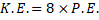Now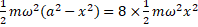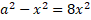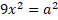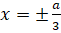Particle will be at a distance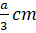from the mean potion on either side.

## FAQs

Q1. Total kinetic energy of simple harmonic motion depends on?

Ans.Total energy is proportional to mass (m), square of frequency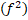and square of amplitude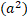Q2. Explain energy in SHM?

Ans. Energy of simple harmonic motion is the sum of kinetic and potential energy at any instant.It remains constant for all times.

Q3. At what potion Kinetic energy and potential energy will be the equal in simple harmonic motion?

Ans. Kinetic energy of a particle is given by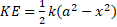Potential energy of particle is given byAccording to question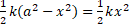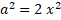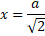So, at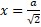KInetic energy and potential energy will be equal in SHM.

Q4. Is the energy of particles executing simple harmonic motion zero at extreme positions?

Ans. No, only kinetic energy is zero.

Q5. What is the type of curve on an energy displacement graph for kinetic and potential energy in simple harmonic motion?

Ans. Energy graph is parabola in shape.Talk to our expert
Resend OTP Timer =
By submitting up, I agree to receive all the Whatsapp communication on my registered number and Aakash terms and conditions and privacy policy